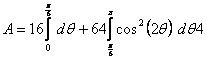Answers to MAT 162 Test 2 Sample Questions

Conic

1.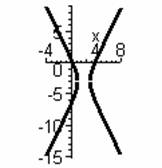Determine what type of conic section is given by the equation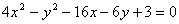.  Put the equation in standard form and identify the important relevant information.  Sketch the curve on the grid below.

Hyperbola

Standard form: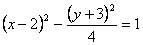center: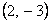vertices: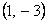,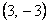foci: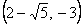,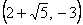Parametric

2.      Below is shown the curve given parametrically by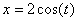,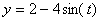over the parameter interval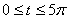. Clearly label the initial point, the terminal point, and the points corresponding to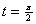,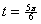and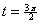. Indicate the direction the curve is traced and how many times the curve is traced (over the interval).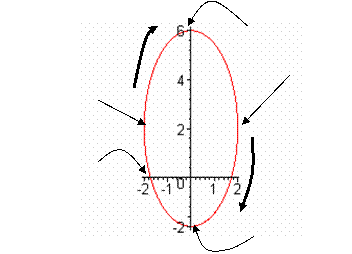3.      Find the Cartesian equation for the curve given in Question #2.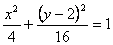4.      Find the derivative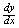and the second derivative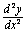of the curve given in Question #2.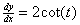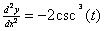5.      Find the (Cartesian) equation of the line tangent to the curve given in Question #2 at the point corresponding to.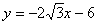6.      Write the integral to find the length of one tracing of the entire curve given in Question #2. (Note: You do not need to evaluate the integral.)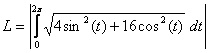7.      Write the integral to find the area inside the curve and below the x-axis, and evaluate this integral.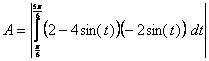8.      Write the integral to find the surface area of the solid generated by revolving the region in Question #7 around the x-axis. (Note: You do not need to evaluate the integral.)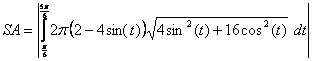9.      Write the integral to find the volume of the solid generated by revolving the region in Question #7 around the x-axis. (Note: You do not need to evaluate the integral.)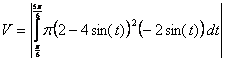## Polar

10.  Plot the point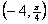on the polar grid below. (Label the initial ray, and include a scale with your plot.) Find two additional representations of this same point, one with r > 0 and one with r < 0. (Note: angles which reduce to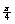are not considered to be different from.)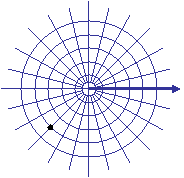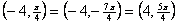Note:  there are many others

11.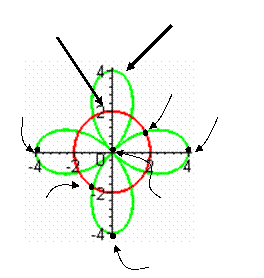Below is shown the curves given by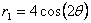and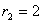.  Identify which curve is which.  Plot and label the points corresponding to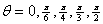and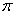on the curve given by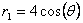.

12.  Find the derivativeof.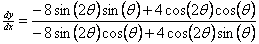13.  Find the (Cartesian) equation of the line tangent to the curve given byat the point where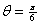.14.  Set up the integral to find the length of one tracing of(Note:  You do not need to evaluate the integral.)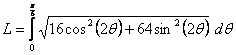15.  Set up the integral to find the area of the region inside the curve given byand outside the curve given by. (Note:  You do not need to evaluate the integral.)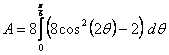16.  Set up the integral to find the area of the region inside both curves (where they overlap)and, and evaluate this integral.